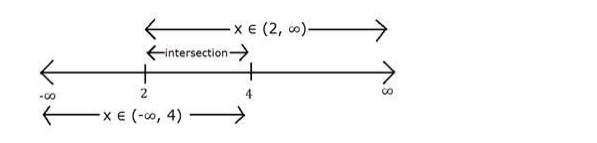# Solve the system of in equation

Question:

Solve the system of in equation $x-2 \geq 0,2 x-5 \leq 3$

Solution:

We have to find values of x for which both the equations hold true

$x-2 \geq 0$ and $2 x-5 \leq 3$

We will solve both the equations separately and then their intersection set will be solution of the system

$x-2 \geq 0$

$\Rightarrow x \geq 2$

Hence $x \in(2, \infty)$

Now, $2 x-5 \leq 3$

$\Rightarrow 2 x \leq 3+5$

$\Rightarrow 2 x \leq 8$

$\Rightarrow x \leq 4$

Hence $x \in(-\infty, 4)$

The intersection of set $(2, \infty)$ and $(-\infty, 4)$ is $(2,4)$

Representing on number lineHence solution set for given system of equation is $x \in(2,4)$